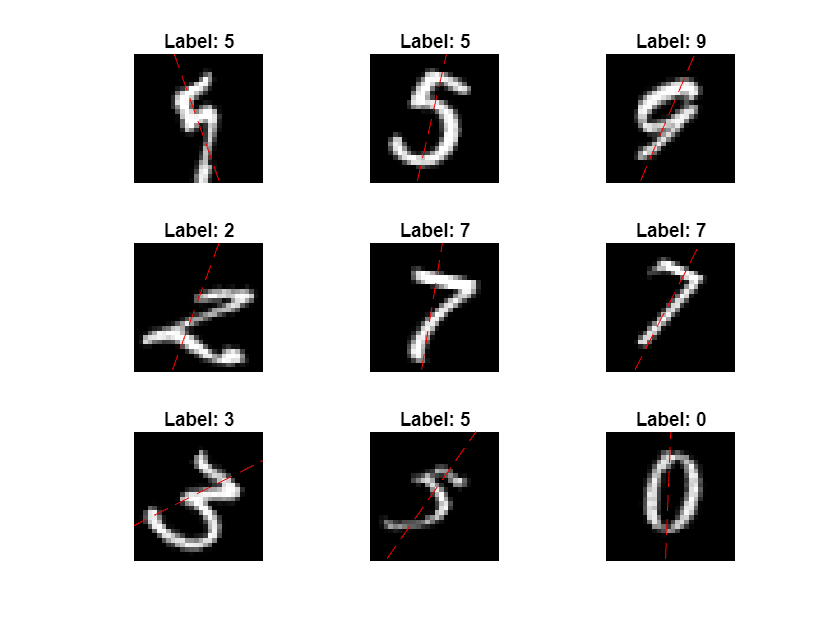# Make Predictions Using Model Function

This example shows how to make predictions using a model function by splitting data into mini-batches.

For large data sets, or when predicting on hardware with limited memory, make predictions by splitting the data into mini-batches. When making predictions with `SeriesNetwork` or `DAGNetwork` objects, the `predict` function automatically splits the input data into mini-batches. For model functions, you must split the data into mini-batches manually.

### Create Model Function and Load Parameters

Load the model parameters from the MAT file `digitsMIMO.mat`. The MAT file contains the model parameters in a struct named `parameters`, the model state in a struct named `state`, and the class names in `classNames`.

```s = load("digitsMIMO.mat"); parameters = s.parameters; state = s.state; classNames = s.classNames;```

The model function `model`, listed at the end of the example, defines the model given the model parameters and state.

Load the digits data for prediction.

```digitDatasetPath = fullfile(matlabroot,'toolbox','nnet','nndemos', ... 'nndatasets','DigitDataset'); imds = imageDatastore(digitDatasetPath, ... 'IncludeSubfolders',true, ... 'LabelSource','foldernames'); numObservations = numel(imds.Files);```

### Make Predictions

Loop over the mini-batches of the test data and make predictions using a custom prediction loop.

Use `minibatchqueue` to process and manage the mini-batches of images. Specify a mini-batch size of 128. Set the read size property of the image datastore to the mini-batch size.

For each mini-batch:

• Use the custom mini-batch preprocessing function `preprocessMiniBatch` (defined at the end of this example) to concatenate the data into a batch and normalize the images.

• Format the images with the dimensions `'SSCB'` (spatial, spatial, channel, batch). By default, the `minibatchqueue` object converts the data to `dlarray` objects with underlying type `single`.

• Make predictions on a GPU if one is available. By default, the `minibatchqueue` object converts the output to a `gpuArray` if a GPU is available. Using a GPU requires Parallel Computing Toolbox™ and a supported GPU device. For information on supported devices, see GPU Computing Requirements (Parallel Computing Toolbox).

```miniBatchSize = 128; imds.ReadSize = miniBatchSize; mbq = minibatchqueue(imds,... "MiniBatchSize",miniBatchSize,... "MiniBatchFcn", @preprocessMiniBatch,... "MiniBatchFormat","SSCB");```

Loop over the minibatches of data and make predictions using the `predict` function. Use the `onehotdecode` function to determine the class labels. Store the predicted class labels.

```doTraining = false; Y1Predictions = []; Y2Predictions = []; % Loop over mini-batches. while hasdata(mbq) % Read mini-batch of data. dlX = next(mbq); % Make predictions using the predict function. [dlY1Pred,dlY2Pred] = model(parameters,dlX,doTraining,state); % Determine corresponding classes. Y1PredBatch = onehotdecode(dlY1Pred,classNames,1); Y1Predictions = [Y1Predictions Y1PredBatch]; Y2PredBatch = extractdata(dlY2Pred); Y2Predictions = [Y2Predictions Y2PredBatch]; end```

View some of the images with their predictions.

```idx = randperm(numObservations,9); figure for i = 1:9 subplot(3,3,i) I = imread(imds.Files{idx(i)}); imshow(I) hold on sz = size(I,1); offset = sz/2; thetaPred = Y2Predictions(idx(i)); plot(offset*[1-tand(thetaPred) 1+tand(thetaPred)],[sz 0],'r--') hold off label = string(Y1Predictions(idx(i))); title("Label: " + label) end```### Model Function

The function `model` takes the model parameters `parameters`, the input data `dlX`, the flag `doTraining` which specifies whether to model should return outputs for training or prediction, and the network state `state`. The network outputs the predictions for the labels, the predictions for the angles, and the updated network state.

```function [dlY1,dlY2,state] = model(parameters,dlX,doTraining,state) % Convolution weights = parameters.conv1.Weights; bias = parameters.conv1.Bias; dlY = dlconv(dlX,weights,bias,'Padding','same'); % Batch normalization, ReLU offset = parameters.batchnorm1.Offset; scale = parameters.batchnorm1.Scale; trainedMean = state.batchnorm1.TrainedMean; trainedVariance = state.batchnorm1.TrainedVariance; if doTraining [dlY,trainedMean,trainedVariance] = batchnorm(dlY,offset,scale,trainedMean,trainedVariance); % Update state state.batchnorm1.TrainedMean = trainedMean; state.batchnorm1.TrainedVariance = trainedVariance; else dlY = batchnorm(dlY,offset,scale,trainedMean,trainedVariance); end dlY = relu(dlY); % Convolution, batch normalization (Skip connection) weights = parameters.convSkip.Weights; bias = parameters.convSkip.Bias; dlYSkip = dlconv(dlY,weights,bias,'Stride',2); offset = parameters.batchnormSkip.Offset; scale = parameters.batchnormSkip.Scale; trainedMean = state.batchnormSkip.TrainedMean; trainedVariance = state.batchnormSkip.TrainedVariance; if doTraining [dlYSkip,trainedMean,trainedVariance] = batchnorm(dlYSkip,offset,scale,trainedMean,trainedVariance); % Update state state.batchnormSkip.TrainedMean = trainedMean; state.batchnormSkip.TrainedVariance = trainedVariance; else dlYSkip = batchnorm(dlYSkip,offset,scale,trainedMean,trainedVariance); end % Convolution weights = parameters.conv2.Weights; bias = parameters.conv2.Bias; dlY = dlconv(dlY,weights,bias,'Padding','same','Stride',2); % Batch normalization, ReLU offset = parameters.batchnorm2.Offset; scale = parameters.batchnorm2.Scale; trainedMean = state.batchnorm2.TrainedMean; trainedVariance = state.batchnorm2.TrainedVariance; if doTraining [dlY,trainedMean,trainedVariance] = batchnorm(dlY,offset,scale,trainedMean,trainedVariance); % Update state state.batchnorm2.TrainedMean = trainedMean; state.batchnorm2.TrainedVariance = trainedVariance; else dlY = batchnorm(dlY,offset,scale,trainedMean,trainedVariance); end dlY = relu(dlY); % Convolution weights = parameters.conv3.Weights; bias = parameters.conv3.Bias; dlY = dlconv(dlY,weights,bias,'Padding','same'); % Batch normalization offset = parameters.batchnorm3.Offset; scale = parameters.batchnorm3.Scale; trainedMean = state.batchnorm3.TrainedMean; trainedVariance = state.batchnorm3.TrainedVariance; if doTraining [dlY,trainedMean,trainedVariance] = batchnorm(dlY,offset,scale,trainedMean,trainedVariance); % Update state state.batchnorm3.TrainedMean = trainedMean; state.batchnorm3.TrainedVariance = trainedVariance; else dlY = batchnorm(dlY,offset,scale,trainedMean,trainedVariance); end % Addition, ReLU dlY = dlYSkip + dlY; dlY = relu(dlY); % Fully connect, softmax (labels) weights = parameters.fc1.Weights; bias = parameters.fc1.Bias; dlY1 = fullyconnect(dlY,weights,bias); dlY1 = softmax(dlY1); % Fully connect (angles) weights = parameters.fc2.Weights; bias = parameters.fc2.Bias; dlY2 = fullyconnect(dlY,weights,bias); end```

### Mini-Batch Preprocessing Function

The `preprocessMiniBatch` function preprocesses the data using the following steps:

1. Extract the data from the incoming cell array and concatenate into a numeric array. Concatenating over the fourth dimension adds a third dimension to each image, to be used as a singleton channel dimension.

2. Normalize the pixel values between `0` and `1`.

```function X = preprocessMiniBatch(data) % Extract image data from cell and concatenate X = cat(4,data{:}); % Normalize the images. X = X/255; end```